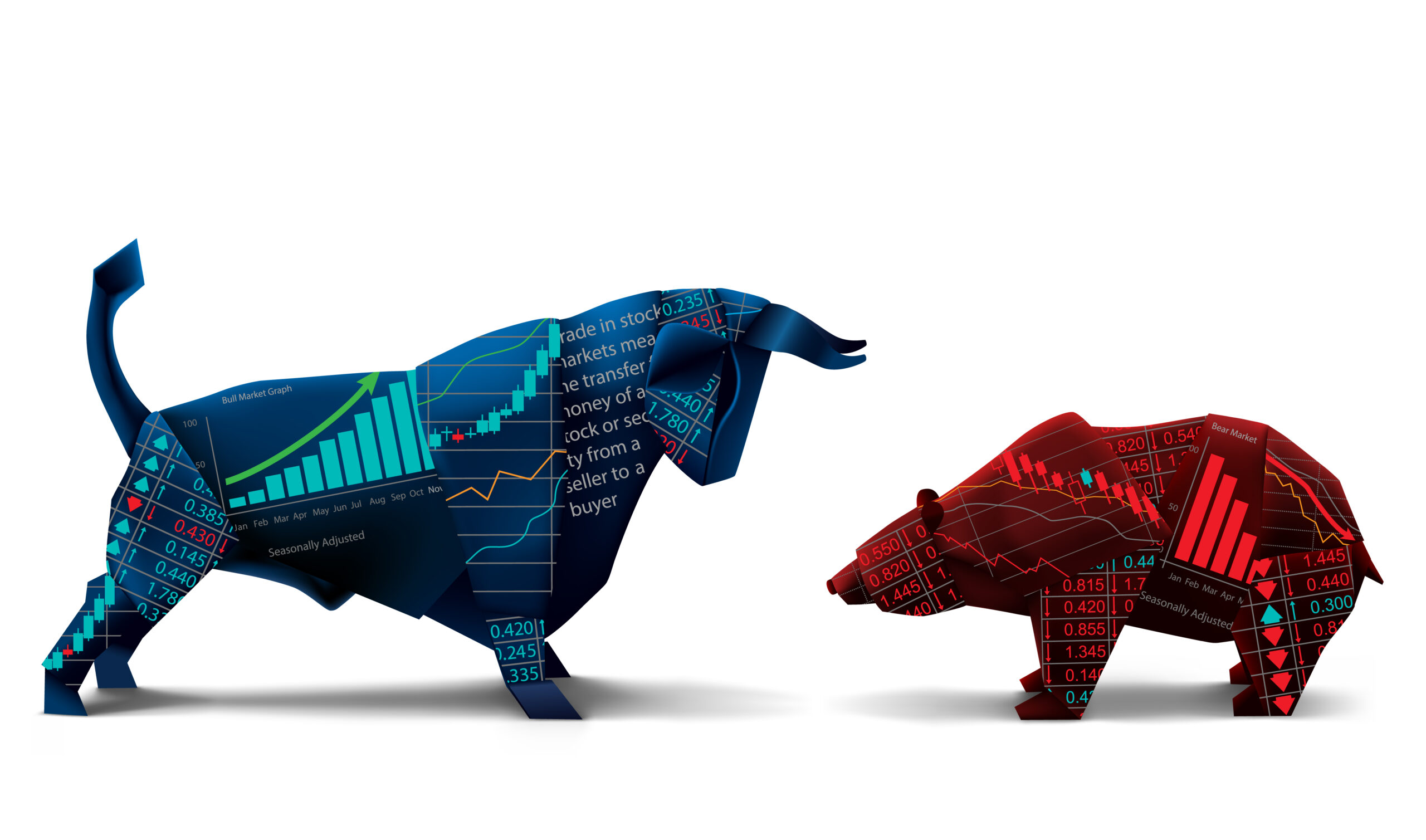# CTM 1.0

## CTM 1.0

This content is protected, please and enroll course to view this content!

1.I think it would really be helpful if this chapter had an elaborate video or maybe if we could have pictures with examples from both platforms to understand the 2 position and 3 position trades.
at the same time showing the 0.5% risk on an actual trade would help understand the risk management concept better.
I personally find this chapter confusing!

1.###### Jordan Lindsey

So important. Good ideas.

2.###### VinceA

I understand the concept and can get behind it, but I’m with Vikram Badri in that I also don’t understand the mathematics involved.
Order 1 = 1x…of what? 1%?? If this were the case, then wouldn’t you be maxing out your risk on the first order?

Example (2 Position Method): 1% just happens to equate to a 1-Lot.
If Order 1 = 1x of my total risk, which ends up being a 1-Lot, then wouldn’t Order 2 = 2x exceed my max risk??

1.###### Jordan Lindsey

1x means lowest size position.
2x is twice as big as the lowest size position.
3x is three times the size of the smallest position.

For example.
0.01 lot or 1000 units
0.02 lots or 2000 units
0.03 lots or 3000 units

3.###### Kyle Shackleford

I too was struggling a bit with the mathematics, PLEASE correct me if I am wrong.
I use Oanda, so figuring out units per trade is easy when placing my stop loss at a 0.5% risk.
So here is my math equation with units

Order 1= 2a
Order 2= 3a
Order 3= a

Order 1= a
Order 2=2a

Hope this is not misinformative and helps

1.###### Jasin Halim

Hi Kyle,
I am using Oanda also and having trouble with MT4 Ea. what i do is for 3 positions, after knowing total positions to enter
p1 = 1/6 * total positions
p2 = 2/6 * total positions
p3 = 3/6 * total positions

Same as for 2 positions
p1 = 1/3 * total positions
p2 = 2/3 * total positions

while keeping the stoploss the same.
Hope this is correct Jordan 🙂

Cheers

1.###### bmangold

Could we just say simply say to get a total of 0.5% risk a 3 position trade would be:

Position 1 = .167% risk
Position 2 = .25% risk
Position 3 = .083% risk

and a 2 position trade would be:
Position 1 = .166% risk
Position 2 = .334% risk

1.###### Jordan Lindsey

Yes, clear. Using Oanda you can simply enter in one trade and exit in pieces for take profits.

2.###### shashamo

Thanks. This is easier and just clear.

4.###### jonnyqwest

this whole segment of the course is confusing. I agree that a video would help to clarify immensely

5.###### kenneth

Should we be taking profits sequentially according to order number? For example in your trades of 3 positions, do you always sell Order 1 = 2x, then Order 2 = 3x, then Order 3 = 1x in that order?

1.###### Jordan Lindsey

I always exit for take profits in this order: 2x then 3x then 1x

SL always the same for each even when moved.

6.###### RichM

I’ve had a light bulb moment on this today. I’d completely missed the concept of multiple trades, I’d been scratching my head on MT4 looking for “how to take a piece off”, I get it now.

7.###### The Balls

Can you please let me know if I have this correct? For clarity sake I am trading Crypto not Forex. So in the below example I am only placing one order and I am going long.

So lets say I have a \$1000 account. At a .05% risk tolerance I should risk \$10. If my trade setup says I should put my stop loss 5% below my buy in price, what is my position size? \$200? Because losing 5% of my \$200 will be a loss of \$10. Is this correct?

1.###### Jordan Lindsey

If you have a \$1,000 account size and risk 0.5% per trade that is a total of \$5 risk per trade. No matter what size your position is (depends on how far away your stop loss) you are risking \$5 per trade. Think in those terms because the position size will vary depending on where your stop loss is from entry.

8.###### jonnyqwest

here is an excellent and easy to understand explanation video of taking a trade off in pieces if you are using Oanda with or without Tradingview. Of course I understand if this doesn’t get posted here since it is another coach’s video.

Select the fields to be shown. Others will be hidden. Drag and drop to rearrange the order.
• Image
• SKU
• Rating
• Price
• Stock
• Availability
• Description
• Content
• Weight
• Dimensions